# 4 Surface energy.ppt

28. May 2023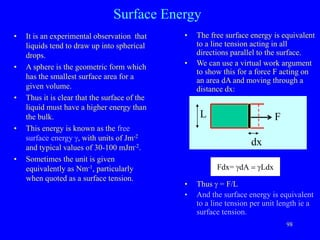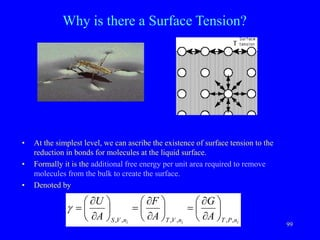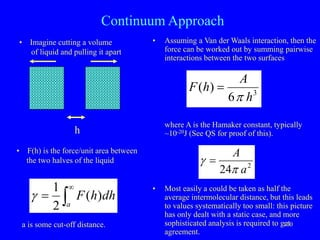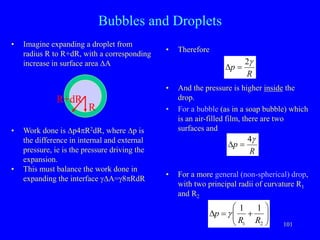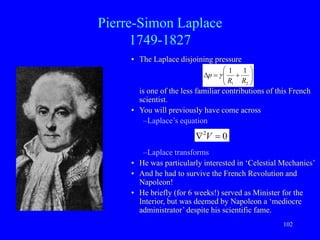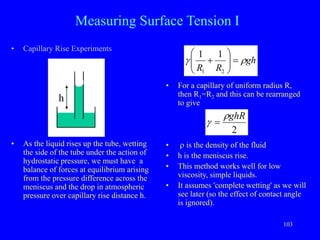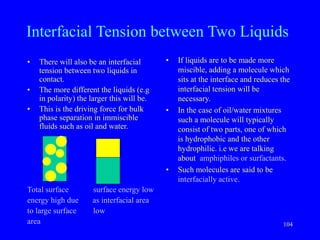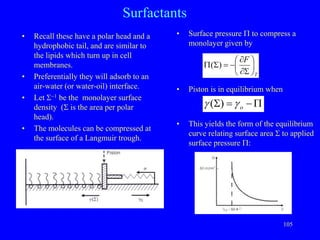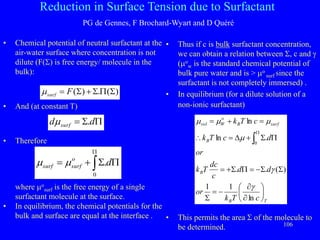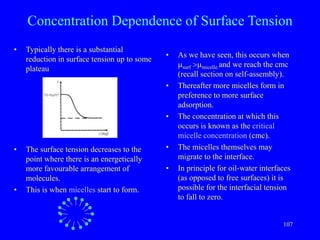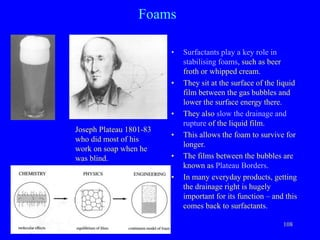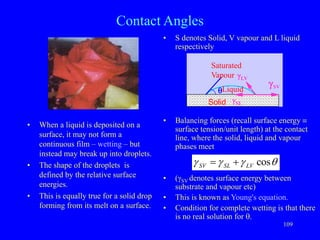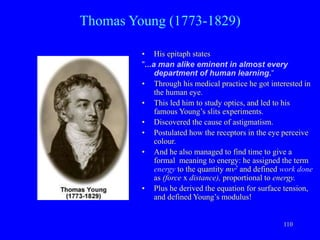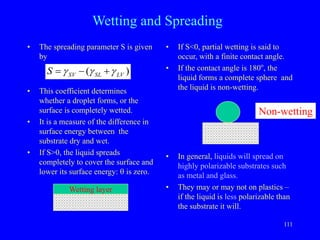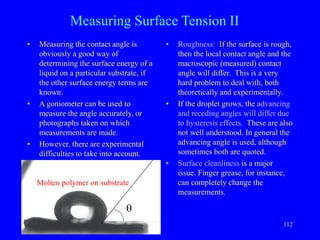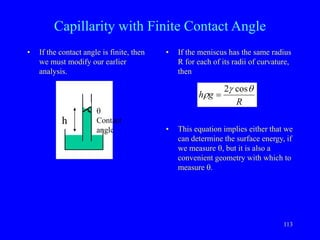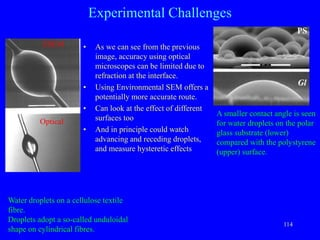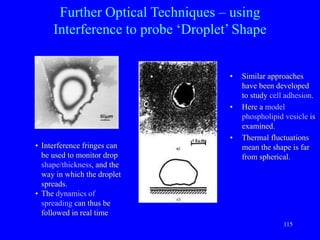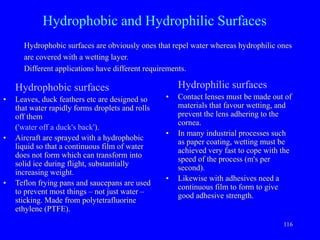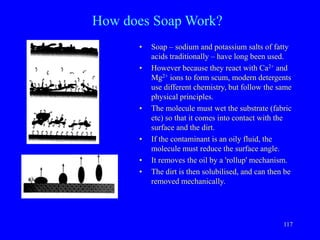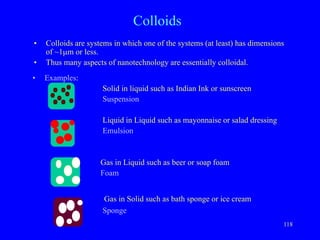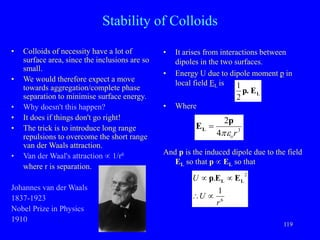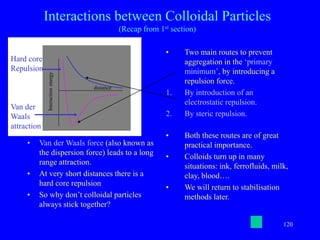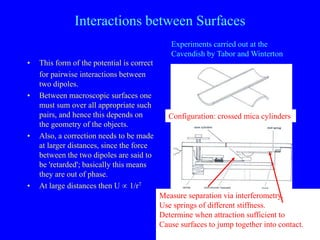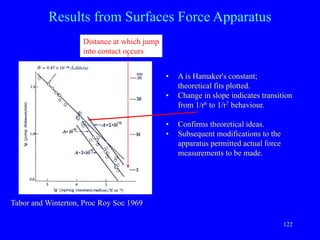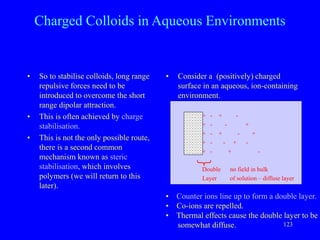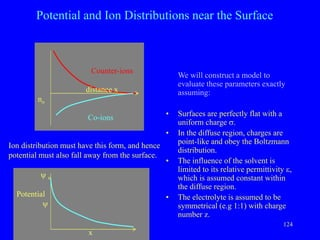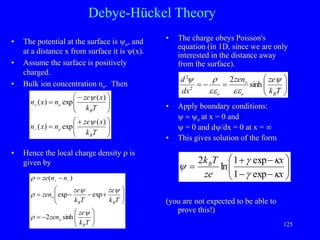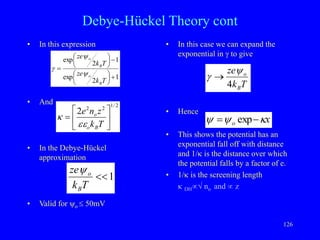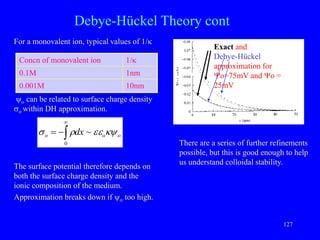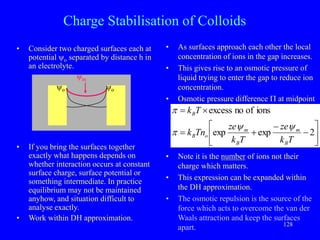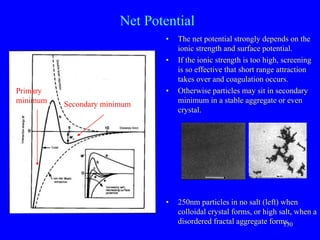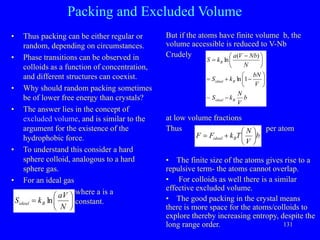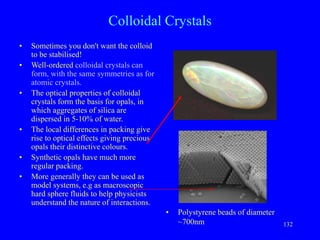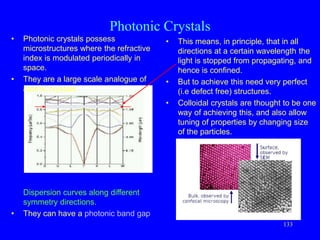1 von 36

### 4 Surface energy.ppt

• 1. 98 Fdx= gdA = gLdx Surface Energy • It is an experimental observation that liquids tend to draw up into spherical drops. • A sphere is the geometric form which has the smallest surface area for a given volume. • Thus it is clear that the surface of the liquid must have a higher energy than the bulk. • This energy is known as the free surface energy g, with units of Jm-2 and typical values of 30-100 mJm-2. • Sometimes the unit is given equivalently as Nm-1, particularly when quoted as a surface tension. • The free surface energy is equivalent to a line tension acting in all directions parallel to the surface. • We can use a virtual work argument to show this for a force F acting on an area dA and moving through a distance dx: • Thus g = F/L • And the surface energy is equivalent to a line tension per unit length ie a surface tension. F L dx
• 2. 99 Why is there a Surface Tension? • At the simplest level, we can ascribe the existence of surface tension to the reduction in bonds for molecules at the liquid surface. • Formally it is the additional free energy per unit area required to remove molecules from the bulk to create the surface. • Denoted by i i i n P T n V T n V S A G A F A U , , , , , ,         =         =         = g
• 3. 100 Continuum Approach • Assuming a Van der Waals interaction, then the force can be worked out by summing pairwise interactions between the two surfaces where A is the Hamaker constant, typically ~10-20J (See QS for proof of this). • Most easily a could be taken as half the average intermolecular distance, but this leads to values systematically too small: this picture has only dealt with a static case, and more sophisticated analysis is required to get agreement. h • F(h) is the force/unit area between the two halves of the liquid   = a dh h F ) ( 2 1 g 3 6 ) ( h A h F  = 2 24 a A  g = • Imagine cutting a volume of liquid and pulling it apart a is some cut-off distance.
• 4. 101 Bubbles and Droplets • Therefore • And the pressure is higher inside the drop. • For a bubble (as in a soap bubble) which is an air-filled film, there are two surfaces and • For a more general (non-spherical) drop, with two principal radii of curvature R1 and R2 • Imagine expanding a droplet from radius R to R+dR, with a corresponding increase in surface area DA • Work done is Dp4R2dR, where Dp is the difference in internal and external pressure, ie is the pressure driving the expansion. • This must balance the work done in expanding the interface gDA=g8RdR R+dR R R p g 2 = D R p g 4 = D          = D 2 1 1 1 R R p g
• 5. 102 Pierre-Simon Laplace 1749-1827 • The Laplace disjoining pressure is one of the less familiar contributions of this French scientist. • You will previously have come across –Laplace’s equation –Laplace transforms • He was particularly interested in ‘Celestial Mechanics’ • And he had to survive the French Revolution and Napoleon! • He briefly (for 6 weeks!) served as Minister for the Interior, but was deemed by Napoleon a ‘mediocre administrator’ despite his scientific fame.          = D 2 1 1 1 R R p g 0 2 =  V
• 6. 103 Measuring Surface Tension I • Capillary Rise Experiments • As the liquid rises up the tube, wetting the side of the tube under the action of hydrostatic pressure, we must have a balance of forces at equilibrium arising from the pressure difference across the meniscus and the drop in atmospheric pressure over capillary rise distance h. • For a capillary of uniform radius R, then R1=R2 and this can be rearranged to give • r is the density of the fluid • h is the meniscus rise. • This method works well for low viscosity, simple liquids. • It assumes 'complete wetting' as we will see later (so the effect of contact angle is ignored). gh R R r g =          2 1 1 1 h 2 ghR r g =
• 7. 104 Interfacial Tension between Two Liquids • There will also be an interfacial tension between two liquids in contact. • The more different the liquids (e.g in polarity) the larger this will be. • This is the driving force for bulk phase separation in immiscible fluids such as oil and water. Total surface surface energy low energy high due as interfacial area to large surface low area • If liquids are to be made more miscible, adding a molecule which sits at the interface and reduces the interfacial tension will be necessary. • In the case of oil/water mixtures such a molecule will typically consist of two parts, one of which is hydrophobic and the other hydrophilic. i.e we are talking about amphiphiles or surfactants. • Such molecules are said to be interfacially active.
• 8. 105 Surfactants • Recall these have a polar head and a hydrophobic tail, and are similar to the lipids which turn up in cell membranes. • Preferentially they will adsorb to an air-water (or water-oil) interface. • Let S-1 be the monolayer surface density (S is the area per polar head). • The molecules can be compressed at the surface of a Langmuir trough. • Surface pressure P to compress a monolayer given by • Piston is in equilibrium when • This yields the form of the equilibrium curve relating surface area S to applied surface pressure P: T F       S   - = S P ) ( P - = S o g g ) (
• 9. 106 Reduction in Surface Tension due to Surfactant PG de Gennes, F Brochard-Wyart and D Quéré • Chemical potential of neutral surfactant at the air-water surface where concentration is not dilute (F(S) is free energy/ molecule in the bulk): • And (at constant T) • Therefore where mo surf is the free energy of a single surfactant molecule at the surface. • In equilibrium, the chemical potentials for the bulk and surface are equal at the interface . • Thus if c is bulk surfactant concentration, we can obtain a relation between S, c and g (mo w is the standard chemical potential of bulk pure water and is > mo surf since the surfactant is not completely immersed) . • In equilibrium (for a dilute solution of a non-ionic surfactant) • This permits the area S of the molecule to be determined. ) ( . ) ( S P S  S = F surf m P S = d d surf . m  P P S  = 0 .d o surf surf m m T B B B surf B o W vol c T k or d d c dc T k or d c T k c T k         - = S S S - = P S  = P S  D =  =  =  P ln 1 1 ) ( . . . ln ln 0 g g m m m m
• 10. 107 Concentration Dependence of Surface Tension • Typically there is a substantial reduction in surface tension up to some plateau • The surface tension decreases to the point where there is an energetically more favourable arrangement of molecules. • This is when micelles start to form. • As we have seen, this occurs when msurf >mmicelle.and we reach the cmc (recall section on self-assembly). • Thereafter more micelles form in preference to more surface adsorption. • The concentration at which this occurs is known as the critical micelle concentration (cmc). • The micelles themselves may migrate to the interface. • In principle for oil-water interfaces (as opposed to free surfaces) it is possible for the interfacial tension to fall to zero.
• 11. 108 Foams • Surfactants play a key role in stabilising foams, such as beer froth or whipped cream. • They sit at the surface of the liquid film between the gas bubbles and lower the surface energy there. • They also slow the drainage and rupture of the liquid film. • This allows the foam to survive for longer. • The films between the bubbles are known as Plateau Borders. • In many everyday products, getting the drainage right is hugely important for its function – and this comes back to surfactants. Joseph Plateau 1801-83 who did most of his work on soap when he was blind.
• 12. 109 Contact Angles • When a liquid is deposited on a surface, it may not form a continuous film – wetting – but instead may break up into droplets. • The shape of the droplets is defined by the relative surface energies. • This is equally true for a solid drop forming from its melt on a surface. • S denotes Solid, V vapour and L liquid respectively • Balancing forces (recall surface energy  surface tension/unit length) at the contact line, where the solid, liquid and vapour phases meet • (gSV denotes surface energy between substrate and vapour etc) • This is known as Young's equation. • Condition for complete wetting is that there is no real solution for q. gLV q g gSL SV Liquid Saturated Vapour Solid q g g g cos LV SL SV  =
• 13. 110 Thomas Young (1773-1829) • His epitaph states "...a man alike eminent in almost every department of human learning.“ • Through his medical practice he got interested in the human eye. • This led him to study optics, and led to his famous Young’s slits experiments. • Discovered the cause of astigmatism. • Postulated how the receptors in the eye perceive colour. • And he also managed to find time to give a formal meaning to energy: he assigned the term energy to the quantity mv2 and defined work done as (force x distance), proportional to energy. • Plus he derived the equation for surface tension, and defined Young’s modulus!
• 14. 111 Wetting and Spreading • The spreading parameter S is given by • This coefficient determines whether a droplet forms, or the surface is completely wetted. • It is a measure of the difference in surface energy between the substrate dry and wet. • If S>0, the liquid spreads completely to cover the surface and lower its surface energy: q is zero. • If S<0, partial wetting is said to occur, with a finite contact angle. • If the contact angle is 180o , the liquid forms a complete sphere and the liquid is non-wetting. • In general, liquids will spread on highly polarizable substrates such as metal and glass. • They may or may not on plastics – if the liquid is less polarizable than the substrate it will. ) ( LV SL SV S g g g  - = Wetting layer Non-wetting
• 15. 112 Measuring Surface Tension II • Measuring the contact angle is obviously a good way of determining the surface energy of a liquid on a particular substrate, if the other surface energy terms are known. • A goniometer can be used to measure the angle accurately, or photographs taken on which measurements are made. • However, there are experimental difficulties to take into account. • Roughness: If the surface is rough, then the local contact angle and the macroscopic (measured) contact angle will differ. This is a very hard problem to deal with, both theoretically and experimentally. • If the droplet grows, the advancing and receding angles will differ due to hysteresis effects. These are also not well understood. In general the advancing angle is used, although sometimes both are quoted. • Surface cleanliness is a major issue. Finger grease, for instance, can completely change the measurements. Molten polymer on substrate q
• 16. 113 Capillarity with Finite Contact Angle • If the meniscus has the same radius R for each of its radii of curvature, then • This equation implies either that we can determine the surface energy, if we measure q, but it is also a convenient geometry with which to measure q. • If the contact angle is finite, then we must modify our earlier analysis. h q Contact angle R g h q g r cos 2 =
• 17. 114 • As we can see from the previous image, accuracy using optical microscopes can be limited due to refraction at the interface. • Using Environmental SEM offers a potentially more accurate route. • Can look at the effect of different surfaces too • And in principle could watch advancing and receding droplets, and measure hysteretic effects Experimental Challenges Optical ESEM Water droplets on a cellulose textile fibre. Droplets adopt a so-called unduloidal shape on cylindrical fibres. A smaller contact angle is seen for water droplets on the polar glass substrate (lower) compared with the polystyrene (upper) surface.
• 18. 115 Further Optical Techniques – using Interference to probe ‘Droplet’ Shape • Similar approaches have been developed to study cell adhesion. • Here a model phospholipid vesicle is examined. • Thermal fluctuations mean the shape is far from spherical. • Interference fringes can be used to monitor drop shape/thickness, and the way in which the droplet spreads. • The dynamics of spreading can thus be followed in real time
• 19. 116 Hydrophobic and Hydrophilic Surfaces Hydrophobic surfaces • Leaves, duck feathers etc are designed so that water rapidly forms droplets and rolls off them ('water off a duck's back'). • Aircraft are sprayed with a hydrophobic liquid so that a continuous film of water does not form which can transform into solid ice during flight, substantially increasing weight. • Teflon frying pans and saucepans are used to prevent most things – not just water – sticking. Made from polytetrafluorine ethylene (PTFE). Hydrophilic surfaces • Contact lenses must be made out of materials that favour wetting, and prevent the lens adhering to the cornea. • In many industrial processes such as paper coating, wetting must be achieved very fast to cope with the speed of the process (m's per second). • Likewise with adhesives need a continuous film to form to give good adhesive strength. Hydrophobic surfaces are obviously ones that repel water whereas hydrophilic ones are covered with a wetting layer. Different applications have different requirements.
• 20. 117 How does Soap Work? • Soap – sodium and potassium salts of fatty acids traditionally – have long been used. • However because they react with Ca2+ and Mg2+ ions to form scum, modern detergents use different chemistry, but follow the same physical principles. • The molecule must wet the substrate (fabric etc) so that it comes into contact with the surface and the dirt. • If the contaminant is an oily fluid, the molecule must reduce the surface angle. • It removes the oil by a 'rollup' mechanism. • The dirt is then solubilised, and can then be removed mechanically.
• 21. 118 Colloids • Colloids are systems in which one of the systems (at least) has dimensions of ~1mm or less. • Thus many aspects of nanotechnology are essentially colloidal. • Examples: Solid in liquid such as Indian Ink or sunscreen Suspension Liquid in Liquid such as mayonnaise or salad dressing Emulsion Gas in Liquid such as beer or soap foam Foam Gas in Solid such as bath sponge or ice cream Sponge
• 22. 119 Stability of Colloids • Colloids of necessity have a lot of surface area, since the inclusions are so small. • We would therefore expect a move towards aggregation/complete phase separation to minimise surface energy. • Why doesn't this happen? • It does if things don't go right! • The trick is to introduce long range repulsions to overcome the short range van der Waals attraction. • Van der Waal's attraction  1/r6 where r is separation. Johannes van der Waals 1837-1923 Nobel Prize in Physics 1910 • It arises from interactions between dipoles in the two surfaces. • Energy U due to dipole moment p in local field EL is • Where And p is the induced dipole due to the field EL so that p  EL so that 3 4 2 r o  p EL = 6 2 1 . r U U     L L E E p L E p. 2 1
• 23. 120 Interactions between Colloidal Particles (Recap from 1st section) • Van der Waals force (also known as the dispersion force) leads to a long range attraction. • At very short distances there is a hard core repulsion • So why don’t colloidal particles always stick together? • Two main routes to prevent aggregation in the ‘primary minimum’, by introducing a repulsion force. 1. By introduction of an electrostatic repulsion. 2. By steric repulsion. • Both these routes are of great practical importance. • Colloids turn up in many situations: ink, ferrofluids, milk, clay, blood…. • We will return to stabilisation methods later. Interaction energy distance Hard core Repulsion Van der Waals attraction
• 24. 121 Interactions between Surfaces • This form of the potential is correct for pairwise interactions between two dipoles. • Between macroscopic surfaces one must sum over all appropriate such pairs, and hence this depends on the geometry of the objects. • Also, a correction needs to be made at larger distances, since the force between the two dipoles are said to be 'retarded'; basically this means they are out of phase. • At large distances then U  1/r7 Experiments carried out at the Cavendish by Tabor and Winterton Configuration: crossed mica cylinders Measure separation via interferometry. Use springs of different stiffness. Determine when attraction sufficient to Cause surfaces to jump together into contact.
• 25. 122 Results from Surfaces Force Apparatus • A is Hamaker's constant; theoretical fits plotted. • Change in slope indicates transition from 1/r6 to 1/r7 behaviour. • Confirms theoretical ideas. • Subsequent modifications to the apparatus permitted actual force measurements to be made. Distance at which jump into contact occurs Tabor and Winterton, Proc Roy Soc 1969
• 26. 123 • Consider a (positively) charged surface in an aqueous, ion-containing environment. + - + - + - - + + - + - + + - - + - + - + - Double no field in bulk Layer of solution – diffuse layer Charged Colloids in Aqueous Environments • So to stabilise colloids, long range repulsive forces need to be introduced to overcome the short range dipolar attraction. • This is often achieved by charge stabilisation. • This is not the only possible route, there is a second common mechanism known as steric stabilisation, which involves polymers (we will return to this later). • Counter ions line up to form a double layer. • Co-ions are repelled. • Thermal effects cause the double layer to be somewhat diffuse.
• 27. 124 Potential and Ion Distributions near the Surface We will construct a model to evaluate these parameters exactly assuming: • Surfaces are perfectly flat with a uniform charge s. • In the diffuse region, charges are point-like and obey the Boltzmann distribution. • The influence of the solvent is limited to its relative permittivity , which is assumed constant within the diffuse region. • The electrolyte is assumed to be symmetrical (e.g 1:1) with charge number z. Counter-ions Co-ions distance x no Ion distribution must have this form, and hence potential must also fall away from the surface.  o Potential  x
• 28. 125 Debye-Hückel Theory • The potential at the surface is o, and at a distance x from surface it is (x). • Assume the surface is positively charged. • Bulk ion concentration no. Then • Hence the local charge density r is given by • The charge obeys Poisson's equation (in 1D, since we are only interested in the distance away from the surface). • Apply boundary conditions:  = o at x = 0 and  = 0 and d/dx = 0 at x =  • This gives solution of the form (you are not expected to be able to prove this!)          =         - = -  T k x ze n x n T k x ze n x n B o B o ) ( exp ) ( ) ( exp ) (           - =          - - = - = -  T k ze zen T k ze T k ze zen n n ze B o B B o  r   r r sinh 2 exp exp ) (         = - = T k ze zen dx d B o o o    r  sinh 2 2 2         - - -  = x x ze T kB  g  g  exp 1 exp 1 ln 2
• 29. 126 Debye-Hückel Theory cont • In this expression • And • In the Debye-Hückel approximation • Valid for o  50mV • In this case we can expand the exponential in g to give • Hence • This shows the potential has an exponential fall off with distance and 1/ is the distance over which the potential falls by a factor of e. • 1/ is the screening length  DH no and  z 1 2 exp 1 2 exp        -       = T k ze T k ze B o B o   g 2 / 1 2 2 2       = T k z n e B o o   1  T k ze B o  T k ze B o 4  g  x o    - = exp
• 30. 127 Debye-Hückel Theory cont For a monovalent ion, typical values of 1/ o can be related to surface charge density so within DH approximation. The surface potential therefore depends on both the surface charge density and the ionic composition of the medium. Approximation breaks down if o too high. Concn of monovalent ion 1/ 0.1M 1nm 0.001M 10nm o o o dx   r s ~ 0   - = There are a series of further refinements possible, but this is good enough to help us understand colloidal stability. Exact and Debye-Hückel approximation for o=75mV and o = 25mV
• 31. 128 Charge Stabilisation of Colloids • Consider two charged surfaces each at potential o separated by distance h in an electrolyte. m o o • If you bring the surfaces together exactly what happens depends on whether interaction occurs at constant surface charge, surface potential or something intermediate. In practice equilibrium may not be maintained anyhow, and situation difficult to analyse exactly. • Work within DH approximation. • As surfaces approach each other the local concentration of ions in the gap increases. • This gives rise to an osmotic pressure of liquid trying to enter the gap to reduce ion concentration. • Osmotic pressure difference P at midpoint • Note it is the number of ions not their charge which matters. • This expression can be expanded within the DH approximation. • The osmotic repulsion is the source of the force which acts to overcome the van der Waals attraction and keep the surfaces apart.       - -  =  = 2 exp exp ions of no excess T k ze T k ze Tn k T k B m B m o B B    
• 32. 129 Charge Stabilisation of Colloids cont • Expand the expression for . • Now m is the sum of two separate potentials at h/2 • So at the midpoint • The case for spherical particles can be similarly treated and results in a similar form for the osmotic pressure ie P  exp-h. (although now the particle radius R also appears in the expression). • The total expression for the net force between two spherical particles becomes attraction repulsion • A is Hamaker's constant, and C is a constant. 2 2 2 ) 2 2 1 1 2 1 1 (         = -         -  -                     = T k ze T k n T k ze T k ze T k ze T k ze T k n B m B o B m B m B m B m B o        2 / exp 2 h o m    - = h T k ze T k n o B B o    -         = exp 4 2 2 h C h AR F  - - = exp 12 2
• 33. 130 Net Potential • The net potential strongly depends on the ionic strength and surface potential. • If the ionic strength is too high, screening is so effective that short range attraction takes over and coagulation occurs. • Otherwise particles may sit in secondary minimum in a stable aggregate or even crystal. • 250nm particles in no salt (left) when colloidal crystal forms, or high salt, when a disordered fractal aggregate forms. Primary minimum Secondary minimum
• 34. 131 Packing and Excluded Volume • Thus packing can be either regular or random, depending on circumstances. • Phase transitions can be observed in colloids as a function of concentration, and different structures can coexist. • Why should random packing sometimes be of lower free energy than crystals? • The answer lies in the concept of excluded volume, and is similar to the argument for the existence of the hydrophobic force. • To understand this consider a hard sphere colloid, analogous to a hard sphere gas. • For an ideal gas where a is a constant. But if the atoms have finite volume b, the volume accessible is reduced to V-Nb Crudely at low volume fractions Thus per atom • The finite size of the atoms gives rise to a repulsive term- the atoms cannot overlap. • For colloids as well there is a similar effective excluded volume. • The good packing in the crystal means there is more space for the atoms/colloids to explore thereby increasing entropy, despite the long range order.       = N aV k S B ideal ln b V N k S V bN k S N Nb V a k S B ideal B ideal B -       -  =       - = ~ 1 ln ) ( ln b V N T k F F B ideal        =
• 35. 132 Colloidal Crystals • Sometimes you don't want the colloid to be stabilised! • Well-ordered colloidal crystals can form, with the same symmetries as for atomic crystals. • The optical properties of colloidal crystals form the basis for opals, in which aggregates of silica are dispersed in 5-10% of water. • The local differences in packing give rise to optical effects giving precious opals their distinctive colours. • Synthetic opals have much more regular packing. • More generally they can be used as model systems, e.g as macroscopic hard sphere fluids to help physicists understand the nature of interactions. • Polystyrene beads of diameter ~700nm
• 36. 133 Photonic Crystals • Photonic crystals possess microstructures where the refractive index is modulated periodically in space. • They are a large scale analogue of atomic crystals Dispersion curves along different symmetry directions. • They can have a photonic band gap • This means, in principle, that in all directions at a certain wavelength the light is stopped from propagating, and hence is confined. • But to achieve this need very perfect (i.e defect free) structures. • Colloidal crystals are thought to be one way of achieving this, and also allow tuning of properties by changing size of the particles.

1. 98
2. 99
3. 100
4. 101
5. 102
6. 103
7. 104
8. 105
9. 106
10. 107
11. 108
12. 109
13. 110
14. 111
15. 112
16. 113
17. 114
18. 115
19. 116
20. 117
21. 118
22. 119
23. 120
24. 121
25. 122
26. 123
27. 124
28. 125
29. 126
30. 127
31. 128
32. 129
33. 130
34. 131
35. 132
36. 133